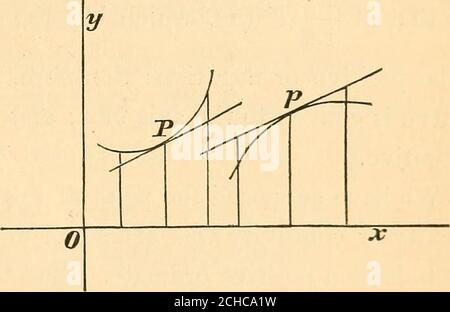RM2CHCA1W. A new treatise on the elements of the differential and integral calculus . towards the line, it is said to be concave, or to have its con-cavity turned towards the line; but, if the sense in which itbends from the tangent is from the line, it is said to be convex,or to have its convexity turned towards the line. To find the conditions of the concavity or convexity of acurve towards a given line, take that line for the axis of x,and let P, of which the co-ordinates are x and y, be the pointat which the curve is to be examined with reference to theseproperties. Draw thetangent at F: then,from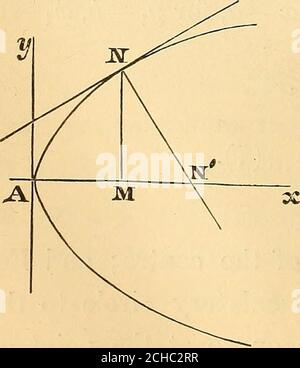RM2CHC2RR. A new treatise on the elements of the differential and integral calculus . d hence that the centre of the circle is always on the concave side of the curve, sincey — 6 is the difference between the ordinate of the point ofcontact and the ordinate of the centre of the osculatory circle.In general, the contact of an osculatory circle is of thesecond order, that is, of an even order; and consequently itcrosses the curve at the point of contact, except at particularpoints where the contact is of an order higher than the second.The osculatory circle is often called circle of curvature; andits cen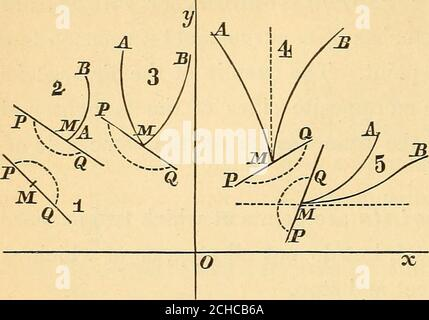RM2CHCB6A. A new treatise on the elements of the differential and integral calculus . n y^ := x^{1 — x^) is that of a curvecomposed of two branches which meet at the origin, and havethe axis of x for a common tangent. The origin is a multiplepoint. Sixth, A point of inflexion is one at which the curveand its tangent at that point cross each other. 151, We will now establish the analytical conditions bywhich the existence and nature of singular points in a curve,if it have any, may be generally recognized; omitting, for thepresent, the case in which the first differential co-efficient ofthe ordinate of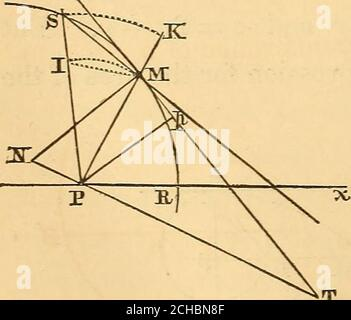RM2CHBN8F. A new treatise on the elements of the differential and integral calculus . D (7, which is measured by -—. But •^ 2 rectangle OLCA = OA X AC = nr X ^r = Inr^^ that is, the rectangle is twice the area of the generating cir-cle : hence 3area semi-cycloid OMGA =: -nr^, and therefore the area bounded by a single branch of thecycloid and its base is three times the area of the generatingcircle; or, in other words, this area is three-fourths of the rec-tangle having for its base the circumference^ and its altitudethe diameter, of the generating circle. Although the area of the cycloid may be said t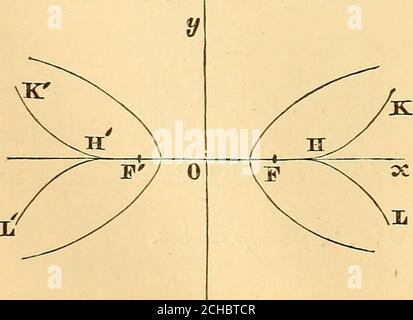RM2CHBTCR. A new treatise on the elements of the differential and integral calculus . 2,|,^ x = -{li —29), y- = 2j)x: whence ?/^ = ^^i^ y-=2^ {22jxy =z 2 )3 and I&gt;^ = 27^ ^^ ^) ^ ^ 27^ (^ -^) for the required equation. If the origin of co-ordinates be transferred to a point atthe distance p in the direction of positive abscissae, the newbeing parallel to the primitive axes, the equation of the evo-lute takes the form 27j9 ^ or V rt We readily recognize that this curve is symmetrical with re-spect to the axis of abscissse, and that it extends without limitin the direction of x positive.By differenti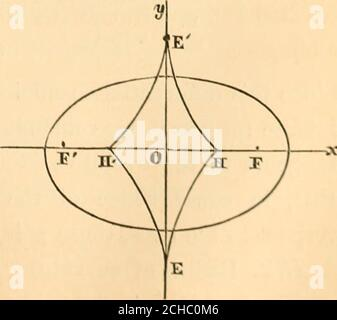RM2CHC0M6. A new treatise on the elements of the differential and integral calculus . ?^) = 0 : EVOLUTE OF THE ELLIPSE. 297 whence y — y = ^ ^ ^ _ ^ i «^: ab y- Making a^ _ ^2 _ ^2^ ^^^ gj^j (5^ + c^?/^)y , c2?/3 c^y^ ,^, 2/-^ = ^-^^-^ =-2/ + -^r- .-.--—/- (3). Substituting in (1) the value of y — ^ just found, we get, afterreduction, and the elimination of y by means of the equation of the ellipse, jm = —^- (4). Eq. 4 might have been derived from (3) by changing the signof the latter, and in it writing x for y, and a for b. This is aconsequence of the symmetry of the equation of the ellipse,and the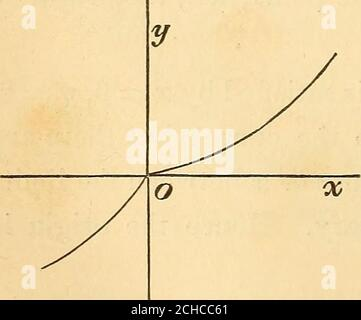RM2CHCC61. A new treatise on the elements of the differential and integral calculus . Ex. 2. y^ie,x. If a; be indefi-nitely great, and positive or nega-tive, y approaches the limit 1; butif X be indefinitely small, and posi-tive, y approaches the limit 0;while, for negative and very small values of a?, y approaches-- oo . The curve will be composed of two branches, as rep-resented in the figure, and will have for the common asymptoteto these the parallel to the axis of x at the distance 1. Third, Points saillant are those at which two branchesof a curve unite and stop, but do not have a common tange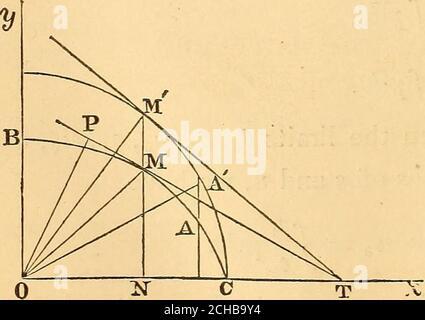RM2CHB9Y4. A new treatise on the elements of the differential and integral calculus . will be positive or negative aeeerding a^^ theangle POx = 0 is greater or less than the aiiuie JlO.v. These 432 INTEGRAL CALCULUS. results may be used for several purposes^ the most importantof which are, — First, To find the length of any portion of a curve, theequation of the curve being given. In this case, from the equation of the curve and the equation dy __dx — cot.^, X and ?/, and therefore ^:) = ic cos. d -- y sin. d, can be determined interms of d; and, by integration, s may be found from the equation s = -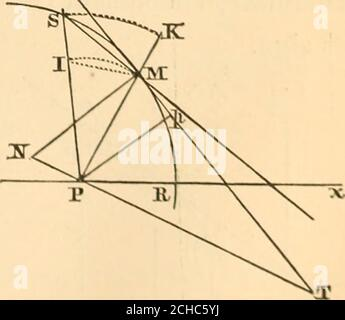RM2CHC5YJ. A new treatise on the elements of the differential and integral calculus . o — a: but 0z=O gives r ^oo ; whence the line parallel to the polar axis at the distance from it equal to — a is an asymptote to the curve. This curve, beginning atan infinite distance, contin-ually approaches the pole,making an indefinite num-ber of turns around withoutever reaching it. 160* When the curve in the vicinity of a tangent line atany point, and the pole, lie on the same side of the tangent,the curve at that point is concave to the pole; but, if thecurve and the pole lie on opposite sides of the tangent, t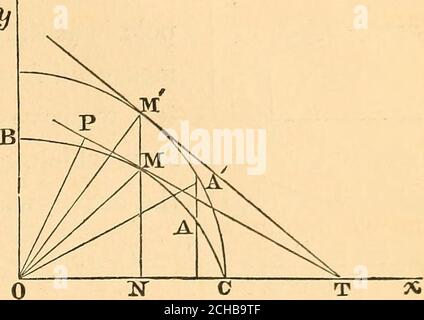RM2CHB9TF. A new treatise on the elements of the differential and integral calculus . dx dt dt and we may have or or -/1(S)*+(S)+(S)T- 254,, To convert the formulae of the last article into polarformulae, take the pole at the origin of co-ordinates, and denoteby d the angle that the radius vector makes with the axis of2, and by g) the angle that its projection on the plane (cc, y)makes with the axis of x; then we have the relationsx^^r sin. 6 cos. (p, y ^r sin. d sin. gy, s = r cos. 6. These three equations, together with the two equations ofthe curve, make five between which we may conceive r and qo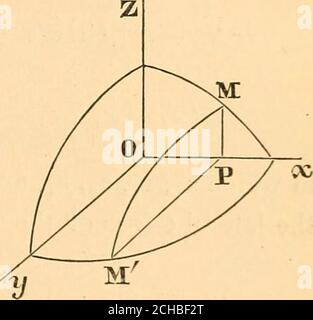RM2CHBF2T. A new treatise on the elements of the differential and integral calculus . ^ the revolution of a single branch of the cycloid, Ex. 5. Volume of an Ellipsoid, Take for the co-ordinate axes the principal axes of the ellipsoid. The equation of its surface is then -^ -^t^—^ = 1.a^ b^ c^ The section PMM of the ellipsoid made by a plane parallelto the plane ZOy, and at the dis-tance OP = X from the origin, hasfor its equation y 2 _ X^ h^ c- a The semi-axes of this section willbe found by making in succession2 :z^ 0, 2/ = 0 ; they are. N a- a- hence the area of the section is ^ a- a- and, for t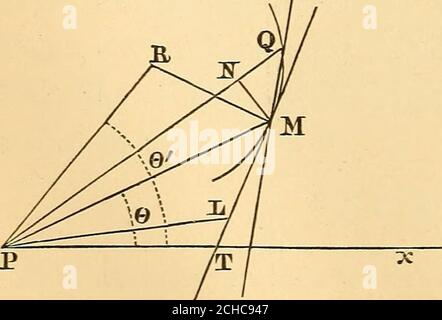RM2CHC947. A new treatise on the elements of the differential and integral calculus . AREAS OF PLANE CURVES. — OF SOLIDS AND SURFACES OFREVOLUTION. 156* Let the pole coincide with the origin of a system ofrectangular co-ordinate axes: denote the radius vector by r,and the angle, called vectorial angle, that it makes with theaxis of X taken as the initial line, or polar axis, by 6; thenthe formulas by which an equation expressed in terms of rec-tangular co-ordinates may be transformed into one expressedin terms of polar co-ordinates are x:=r cos. 6, y ^=.r sin. d. To express in polar cO-ordinates the ta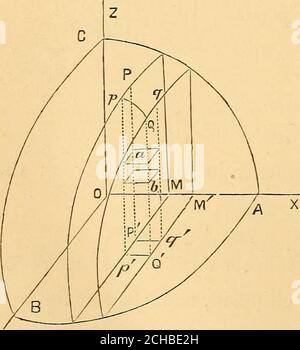RM2CHBE2H. A new treatise on the elements of the differential and integral calculus . huno 64 426 INTEGRAL CALCULUS, Ex. 6. The areas of surfaces and volumes of solids havethus far been found by single integration. As an example ofdouble integration, let it be required to find the volumebounded by the surface determined by the equation xy ziz az,and by the four planes having for their equations x = x^, x=x,^, y — Vi, y — Vi.The expression for this volume is =/:;/;;f**=r.w-=5(:-&lt;) = J {^2 - ^1) {y-i — ^1) (2i + 2., + ^3 + 24). in which z^, 29; ^3? ^4? ^^^ the ordinates of the points in which the late